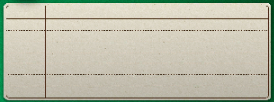# A qualitative change size of the BitmapData in webGL. How?

Hello everybody.

I’m developing an application on openFL html5.
It works slowly.
If only just to display one image at full screen processor is fully loaded and the frame rate is not high.
If something more complicated one picture is even worse.

I’m trying to make it work faster but facing difficulties and I ask to help me.

I’m tuning my application rendering speed.

Now I’m trying to make a preliminary image resizing. To no change in image size and painting is faster at copying.
I tried to do this with BitmapData.draw but the picture quality is obtained in this way is terrible. Moreover, it does not work in webGLI wrote the quality resizing function.
I did a lot of shaman dances that it can work.

``````public static function downscaleImage(imageBitmapData:BitmapData, W2:Int, H2:Int):BitmapData {

var newBitmap = new BitmapData(W2, H2, true);
var rect = new Rectangle(0,0,W2, H2);
if (imageBitmapData == null) return newBitmap;

lime.graphics.utils.ImageCanvasUtil.convertToCanvas (imageBitmapData.image);

var W = imageBitmapData.width;
var H = imageBitmapData.height;

var img = imageBitmapData.image.buffer.__srcCanvas.getContext2d().getImageData(0,0,W,H);

var data =  img.data;
var data2 =  newBitmap.getPixels(rect);

var ratio_w = W / W2;
var ratio_h = H / H2;
var ratio_w_half = Math.ceil(ratio_w/2);
var ratio_h_half = Math.ceil(ratio_h/2);

for (j in 0...H2) {
for (i in 0...W2) {
var x2 = (i + j * W2) * 4;
var weight = 0.0;
var weights = 0.0;
var weights_alpha = 0.0;
var gx_r, gx_g, gx_b, gx_a;
gx_r = gx_g = gx_b = gx_a = 0.0;
var center_y = (j + 0.5) * ratio_h;
for (yy in Math.floor(j * ratio_h) ... Math.ceil((j + 1) * ratio_h)) {
var dy = Math.abs(center_y - (yy + 0.5)) / ratio_h_half;
var center_x = (i + 0.5) * ratio_w;
var w0 = dy * dy; //pre-calc part of w
for (xx in Math.floor(i * ratio_w)...Math.ceil((i + 1) * ratio_w)) {
var dx0 = Math.abs(center_x - (xx + 0.5)) / ratio_w_half;
var w = Math.sqrt(w0 + dx0 * dx0);
if (w >= -1 && w <= 1) {
//hermite filter
weight = 2 * w * w * w - 3 * w * w + 1;
if (weight > 0) {
var dx = 4 * (xx + yy * W);
//alpha
gx_a += weight * data[dx + 3];
weights_alpha += weight;
//colors
if (data[dx + 3] < 255)
weight = weight * data[dx + 3] / 250;
gx_r += weight * data[dx];
gx_g += weight * data[dx + 1];
gx_b += weight * data[dx + 2];
weights += weight;
}
}
}
}
data2[x2] = Std.int(gx_a / weights_alpha);
data2[x2 + 1] = Std.int(gx_r / weights);
data2[x2 + 2] = Std.int(gx_g / weights);
data2[x2 + 3] = Std.int(gx_b / weights);

}
}
newBitmap.setPixels(rect, data2);
return newBitmap;
}
``````

It work for webgl. This function is not very fast, but prerendered graphics renders faster.

But for some reason this does not work for the BitmapData derived from spritesheet in webGLHow does this win??
Why this can happen?
I create bitmap flooded with color. With it, this function works.
Spritesheet uses copyPixels. Thereafter in webGL my function does not work.
I tried to replace copyPixels to getPixels/setPixels, but it does not work.

How to fix it?

What are you trying to accomplish?

Can you use `scaleX`/`scaleY` to shrink down your image?

If you must use a method to resize, can you use `bitmapData.draw`?

Sorry for my bad English… I try to translate as best I can.

If I use scaleX/scaleY performance falls. In this case, when repainting occurs every frame resizing.

bitmapData.draw gives a very poor quality. The image below is the result bitmapData.draw. Many lines are missing.Does `scaleX` plus using `openfl test html5 -Dwebgl` help?

Oh it looks good, but slow.

On my computer, any option is fast enough))
But I program not only for themselves.

On slower computers and mobile phone even in webgl mode, there is a big difference in performance. Between prescaled graphics and not prescaled.

It would be really cool if we could do a redraw only the changed parts of the screen. How this is done in a flash. It was a very accelerated redraw in many cases.
But I really have no idea how to do it in openfl(html5).

How often do you need to resize? Can you resize the asset once (using `bitmapData.draw`) then use it unscaled from then on, or do you need to constantly modify at a larger scale?

Resize asset is rarely needed. When you start the application under the current screen size. And when you change the size of the screen (such as a smartphone screen rotation). If the sprite is often changes in the size of the course for him to make rational scaleX/Y.

How big is your image and how many of those are you drawing each frame?